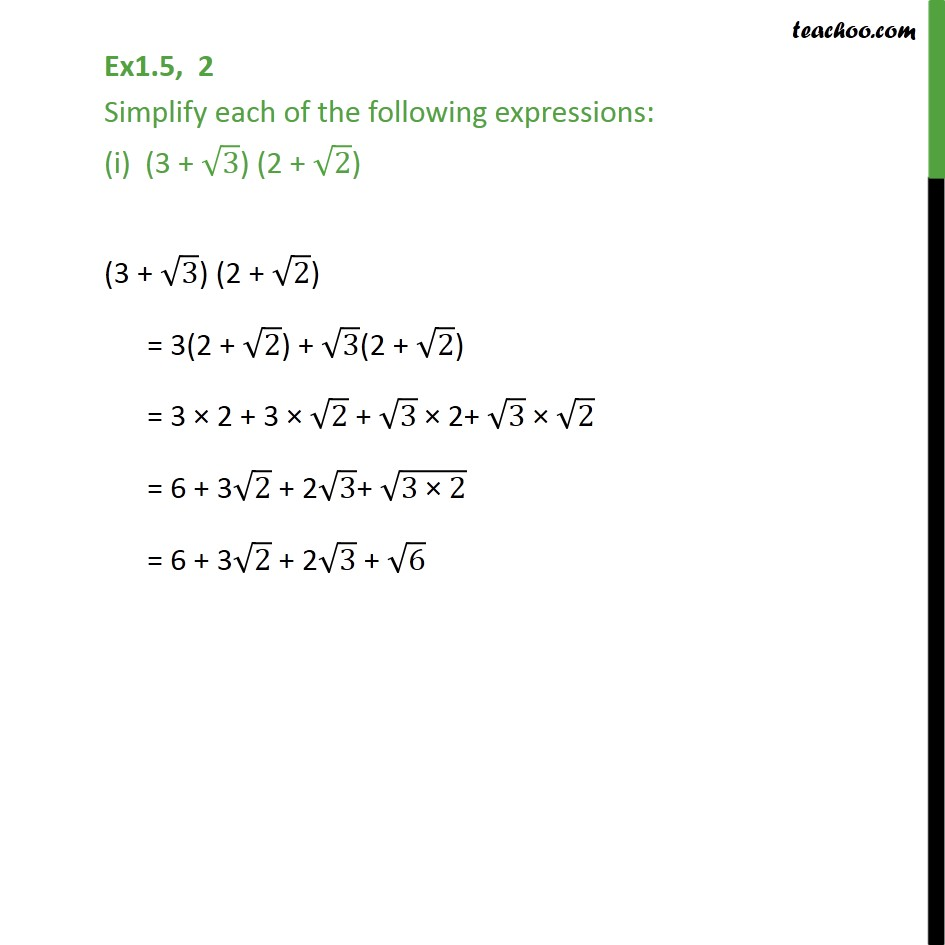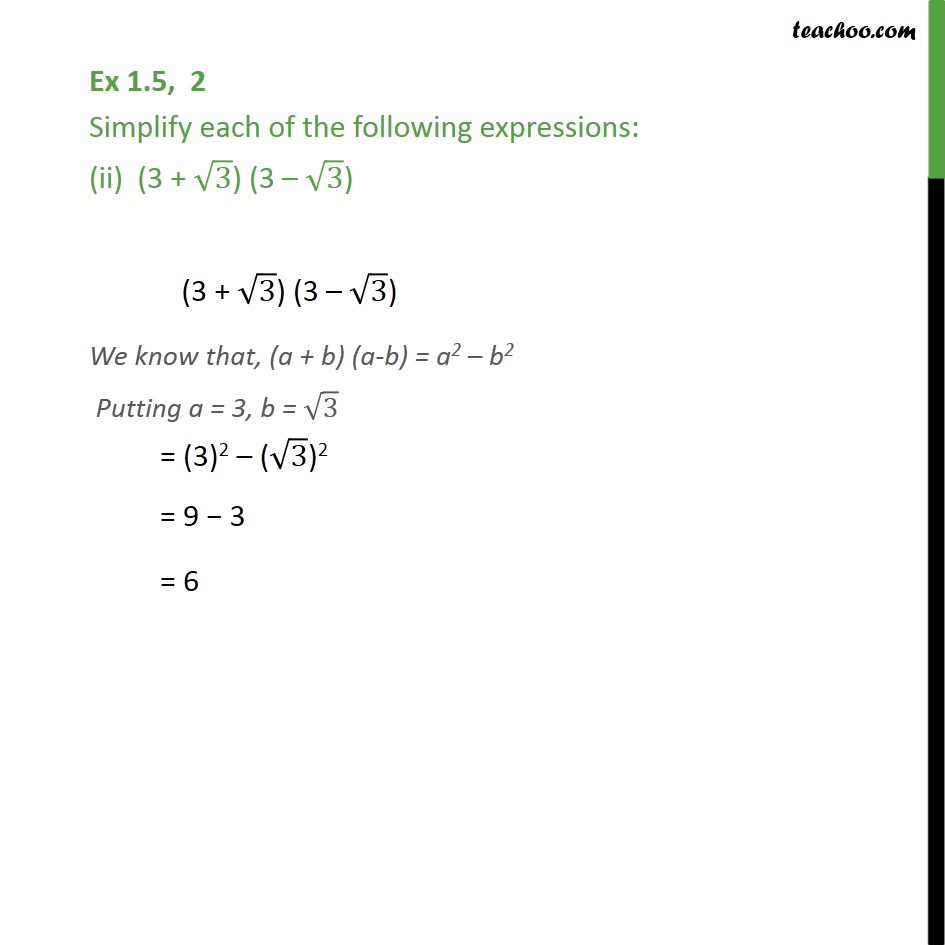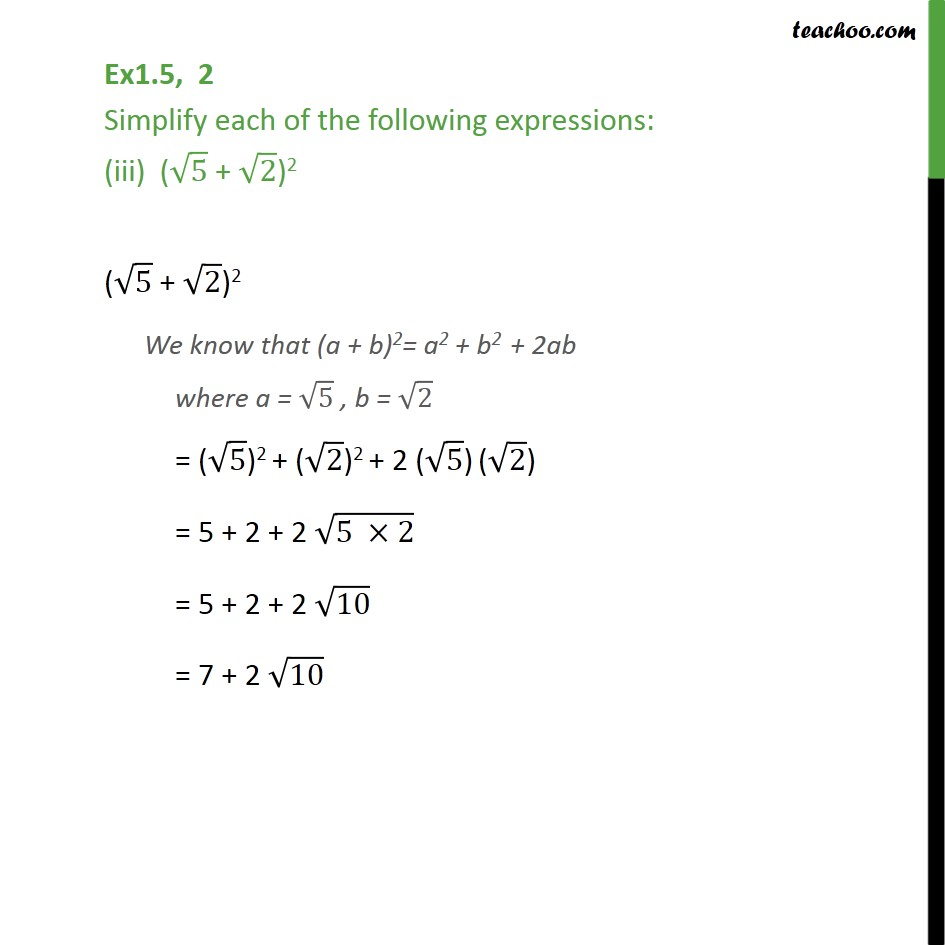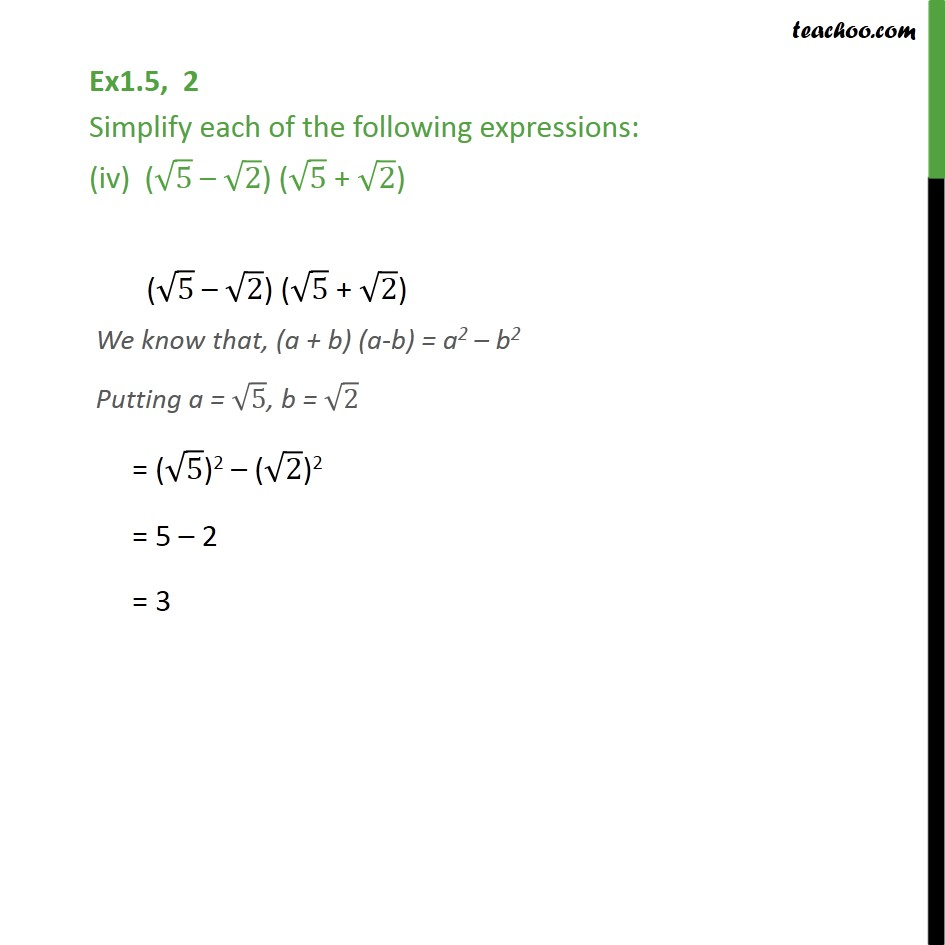1. Chapter 1 Class 9 Number Systems (Term 1)
2. Concept wise
3. Simplifying real numbers

Transcript

Ex1.5, 2 Simplify each of the following expressions: (i) (3 + 3) (2 + 2) (3 + 3) (2 + 2) = 3(2 + 2) + 3(2 + 2) = 3 2 + 3 2 + 3 2+ 3 2 = 6 + 3 2 + 2 3+ (3" " 2) = 6 + 3 2 + 2 3 + 6 Ex 1.5, 2 Simplify each of the following expressions: (ii) (3 + 3) (3 3) (3 + 3) (3 3) We know that, (a + b) (a-b) = a2 b2 Putting a = 3, b = 3 = (3)2 ( 3)2 = 9 3 = 6 Ex1.5, 2 Simplify each of the following expressions: (iii) ( 5 + 2)2 ( 5 + 2)2 We know that (a + b)2= a2 + b2 + 2ab where a = 5 , b = 2 = ( 5)2 + ( 2)2 + 2 ( 5) ( 2) = 5 + 2 + 2 (5 2) = 5 + 2 + 2 10 = 7 + 2 10 Ex1.5, 2 Simplify each of the following expressions: (iv) ( 5 2) ( 5 + 2) ( 5 2) ( 5 + 2) We know that, (a + b) (a-b) = a2 b2 Putting a = 5, b = 2 = ( 5)2 ( 2)2 = 5 2 = 3

Simplifying real numbers